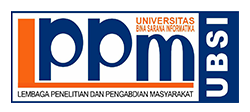### THE CORRELATION BETWEEN STUDENTS VOCABULARY ACHIEVEMENT AND STUDENTS READING COMPREHENSION ACHIEVEMENT AT THE SECOND GRADE OF SMPN 30 BEKASI

Ferawaty Puspitorini

#### Abstract

In this research there are two variables: the independent variable is students vocabulary achievement and the dependent variable is students reading comprehension achievement. The purpose of this research are to find out whether there is correlation or not between students vocabulary achievement and students reading comprehension achievement at the second grade students of SMPN 30 Bekasi.

The research method is ex post facto. The research is conducted all the second grade students of SMPN 30 Bekasi. The population of research is the total numbers of students are 272 students there are 6 classes in the second grade. The students are given post test.

The sampling technique is simple random sampling. The result of data analysis through correlation Pearson Product Moment which has been modification,  = 23,806 if compared by . Value criticize of chi square for the df (degree of freedom) equal to 1, level significant 0,05, =  3,841. It means that >.

So, from the hypothesis we can see that Ha is accepted and H0 is rejected. Based on the result from the interpretation the research concludes that there is significant The Correlation between students Vocabulary Achievement and Students Reading Comprehension Achievement at the Second Grade at SMPN 30 Bekasi.

Keywords: Vocabulary, Vocabulary Achievement, Reading Comprehension

#### Full Text:

PDF

DOI: https://doi.org/10.31294/w.v9i1.1316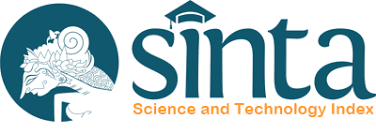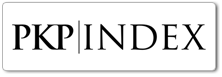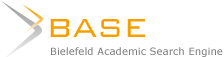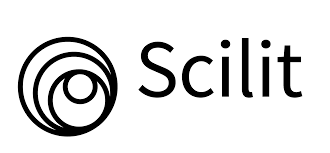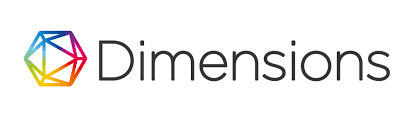####